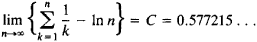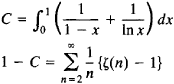# Euler's Constant

Also found in: Wikipedia.

## Euler's constant

[′ȯi·lərz ¦kän·stənt]
(mathematics)
The limit as n approaches infinity, of 1 + 1/2 + 1/3 + ⋯ + 1/ n- ln n, equal to approximately 0.5772. Denoted γ. Also known as Mascheroni's constant.
McGraw-Hill Dictionary of Scientific & Technical Terms, 6E, Copyright © 2003 by The McGraw-Hill Companies, Inc.
The following article is from The Great Soviet Encyclopedia (1979). It might be outdated or ideologically biased.

## Euler’s Constant

(or Mascheroni’s constant), the limitwhich was considered by L. Euler in 1740. Euler gave a number of representations for C in the form of series and integrals; for example,where ζ(s) is the zeta function. Euler’s constant is encountered in the theory of various classes of special functions, such as the gamma function. It remains unknown whether Euler’s constant is an irrational number.

Mentioned in ?
References in periodicals archive ?
This is called a harmonic series and it can be shown that the sum is of the order: n(ln(n) + Y) (where Y = Euler's constant = 0.557) where n is the number of stickers required to collect.
Euler's constant 1/(lim(x-->infinity) Euler's constant ((1-(1/x))^x)) W^(k/S) Euler's constant [W=number of microstates; k=Boltzmann constant; S=entropy] F/[N.sub.A] Elementary charge [F=Faraday constant; NA Avogadro constant] 1.6022*(10^-19)(C) Elementary charge F ma Force [m=mass; a=acceleration] v Frequency of a wave [v=frequency] v/lambda] Frequency of a wave [v=velocity; [lambda]=wavelength] c/[lambda] Frequency of a wave in a vacuum [c=speed of light; [lambda]=wavelength] E/h Frequency of a wave [E=energy; h=Planck's constant] e[N.sub.A] Faraday constant [[N.sub.A]=Avogadro constant; e=elementary charge] 96485.3365 ...
Mortici, Optimizing the rate of convergence in some new classes of sequences convergent to Euler's constant, Anal.
Sandor, Double integrals for Euler's constant and ln 4/[pi] and an analog of Hadjicosta's formula.
Some third of the material is concerned with biographical and other contextual issues, while the bulk of the selections focus on particular aspects of Euler's contributions to mathematics, including infinite series, the zeta functions, Euler's constant, differentials, multiple integrals, the calculus of variations, the pentagonal number theorem, quadratic reciprocity, and the fundamental theorem of algebra.
We note that the function on the left-hand side of (1) is equal to [e.sup.-[gamma]x]/[GAMMA] (1 + x), where [gamma] is Euler's constant and [GAMMA](x) is the gamma function.
denotes the Euler's constant, which is equivalent to [[[GAMMA](1 + x)].sup.1/x]/[x.sup.1-[gamma]] being increasing on (1, [infinity]).
[gamma] is the Euler's constant, [MATHEMATICAL EXPRESSION NOT REPRODUCIBLE IN ASCII] denotes the product over all primes.
As an application, we give the upper and lower bounds for the expression [n.summation over (k=1)] 1/k - 1n n - [gamma] = 0.57721 x x x is the Euler's constant.
where [gamma] is the Euler's constant. Now combining the Stirling Formula (see reference ), we can get

Site: Follow: Share:
Open / Close